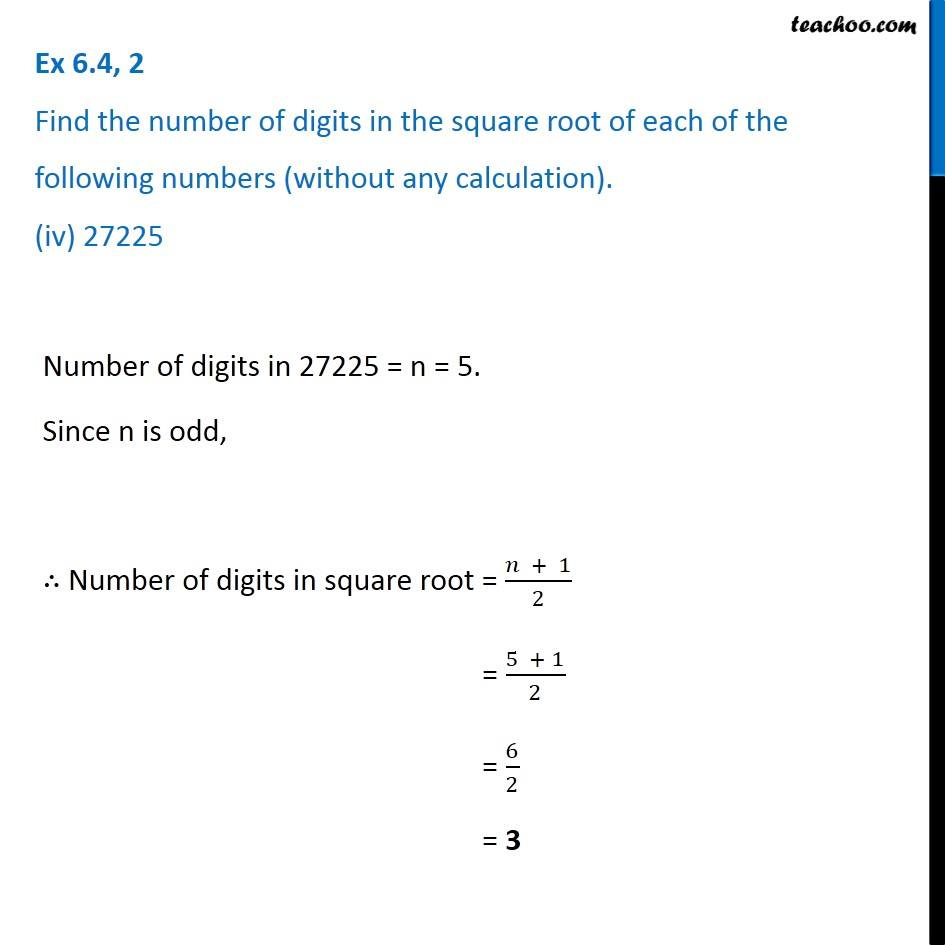Ex 6.4

Chapter 6 Class 8 Squares and Square Roots
Serial order wiseIntroducing your new favourite teacher - Teachoo Black, at only ₹83 per month

### Transcript

Ex 6.4, 2 Find the number of digits in the square root of each of the following numbers (without any calculation). (iv) 27225 Number of digits in 27225 = n = 5. Since n is odd, ∴ Number of digits in square root = (𝑛 + 1)/2 = (5 + 1)/2 = 6/2 = 3